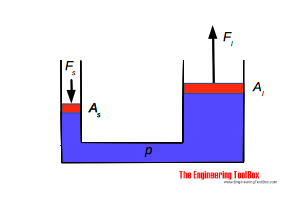Engineering ToolBox - Resources, Tools and Basic Information for Engineering and Design of Technical Applications!

# Hydraulic Force vs. Pascal's Law

## Pascal's law and the hydraulic force acting in fluids.

Pascal's Laws relates to pressures in incompressible fluids - liquids.

• if the weight of a fluid is neglected the pressure throughout an enclosed volume will be the same
• the static pressure in a fluid acts equally in all directions
• the static pressure acts at right angles to any surface in contact with the fluid

### Example - Pressure in a Hydraulic Cylinder

The pressure of 2000 Pa in an hydraulic cylinder acts equally on all surfaces. The force on a piston with area 0.1 m2 can be calculated

F = p A                              (1)

where

F = force (N)

p = pressure (Pa, N/m2)

A = area (m2)

or with values

F = (2000 Pa) (0.1 m2)

= 200 (N)

### Example - Force in a Hydraulic JackThe pressure acting on both pistons in a hydraulic jack is equal.The force equation for the small cylinder:

Fs = p As                              (2)

where

Fs = force acting on the piston in the small cylinder (N)

As = area of small cylinder (m2)

p = pressure in small and large cylinder  (Pa, N/m2)

The force equation for the large cylinder:

Fl = p Al                             (2b)

where

Fl = force acting on the piston in the large cylinder (N)

Al = area of large cylinder (m2)

p = pressure in small and large cylinder (Pa, N/m2)

(2) and (2b) can be combined to

Fs / As = Fl / Al                                (2c)

or

Fs = Fl As / Al                                  (2d)

The equation indicates that the effort force required in the small cylinder to lift a load on the large cylinder depends on the area ratio between the small and the large cylinder - the effort force can be reduced by reducing the small cylinder area compared to the large cylinder area.

### Related Mobile Apps from The Engineering ToolBox- free apps for offline use on mobile devices.

### A Hydraulic Jack Lifting a Car

The back end (half the weight) of a car of mass 2000 kg is lifted by an hydraulic jack where the As / Al ratio is 0.1 (the area of the large cylinder is 10 times the area of the small cylinder).

The force - weight - acting on the large cylinder can be calculated with Newton's Second Law:

Fl = m a

where

m = mass (kg)

a = acceleration of gravity (m/s2)

or

Fl = 1/2 (2000 kg) (9.81 m/s2)

= 9810 (N)

The force acting on the small cylinder in the jack can be calculated with (2d)

Fs = (9810 N) 0.1

= 981 (N)

## Related Topics

• ### Fluid Mechanics

The study of fluids - liquids and gases. Involving velocity, pressure, density and temperature as functions of space and time.
• ### Gases and Compressed Air

Air, LNG, LPG and other common gas properties, pipeline capacities, sizing of relief valves.

## Related Documents

• ### Hydraulic Force vs. Pressure and Cylinder Size

Calculate hydraulic cylinder force.
• ### Hydrostatic Pressure vs. Depth

Depth and hydrostatic pressure.
• ### Pressure

Introduction to pressure - online pressure units converter.

## Engineering ToolBox - SketchUp Extension - Online 3D modeling!

Add standard and customized parametric components - like flange beams, lumbers, piping, stairs and more - to your Sketchup model with the Engineering ToolBox - SketchUp Extension - enabled for use with older versions of the amazing SketchUp Make and the newer "up to date" SketchUp Pro . Add the Engineering ToolBox extension to your SketchUp Make/Pro from the Extension Warehouse !

We don't collect information from our users. More about

## Citation

• The Engineering ToolBox (2008). Hydraulic Force vs. Pascal's Law. [online] Available at: https://www.engineeringtoolbox.com/pascal-laws-d_1274.html [Accessed Day Month Year].

Modify the access date according your visit.

9.19.12